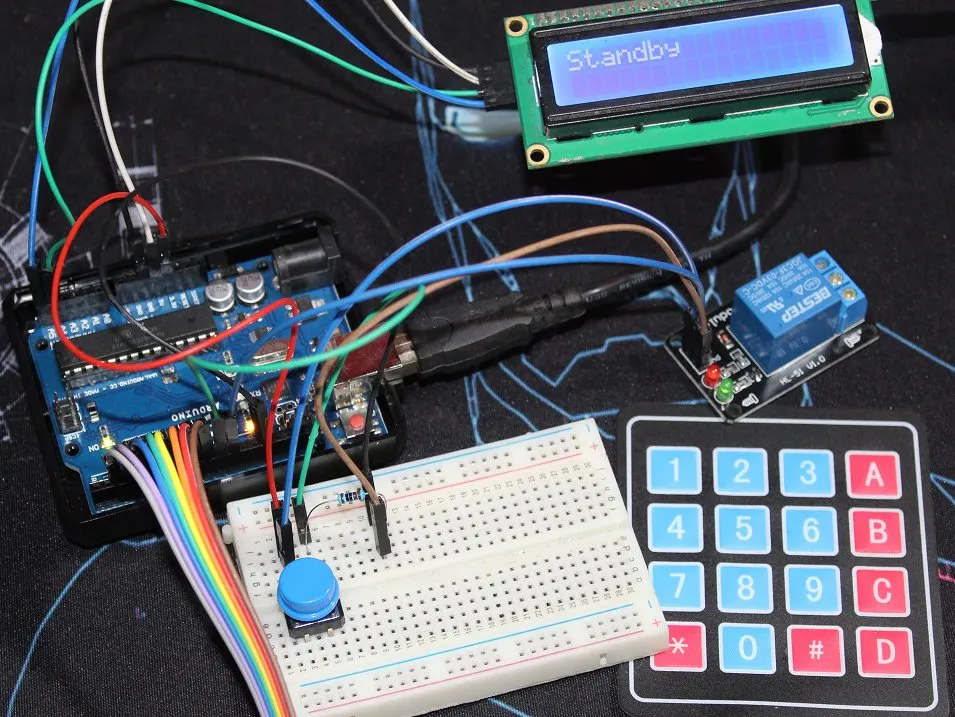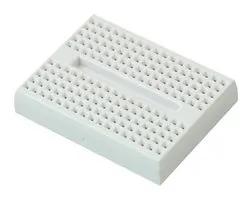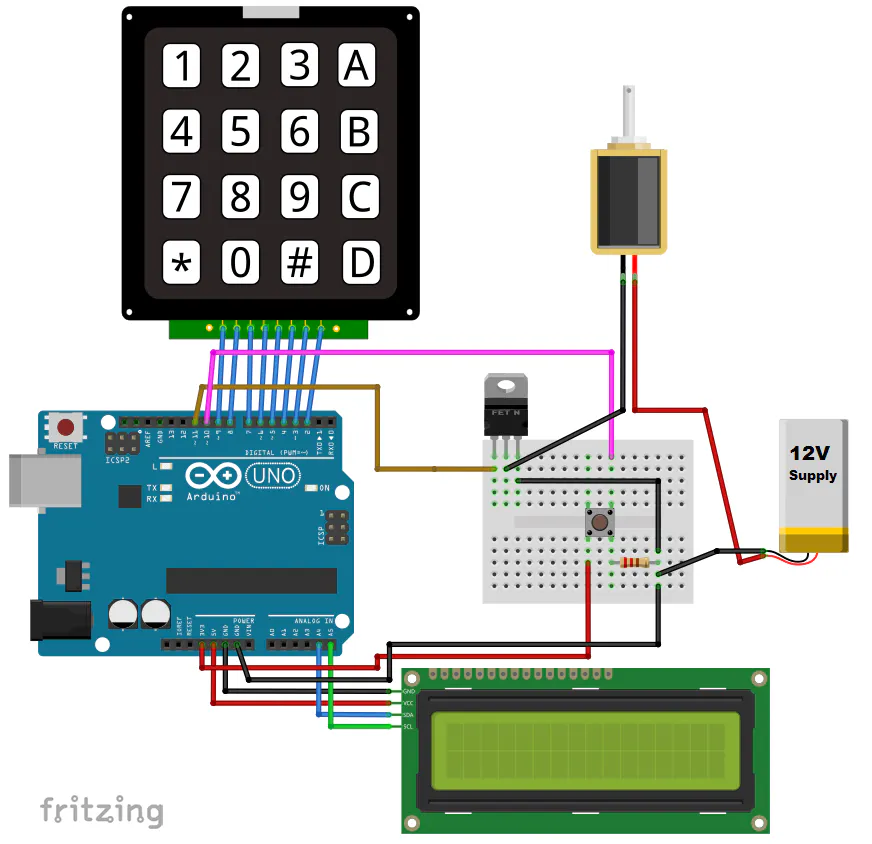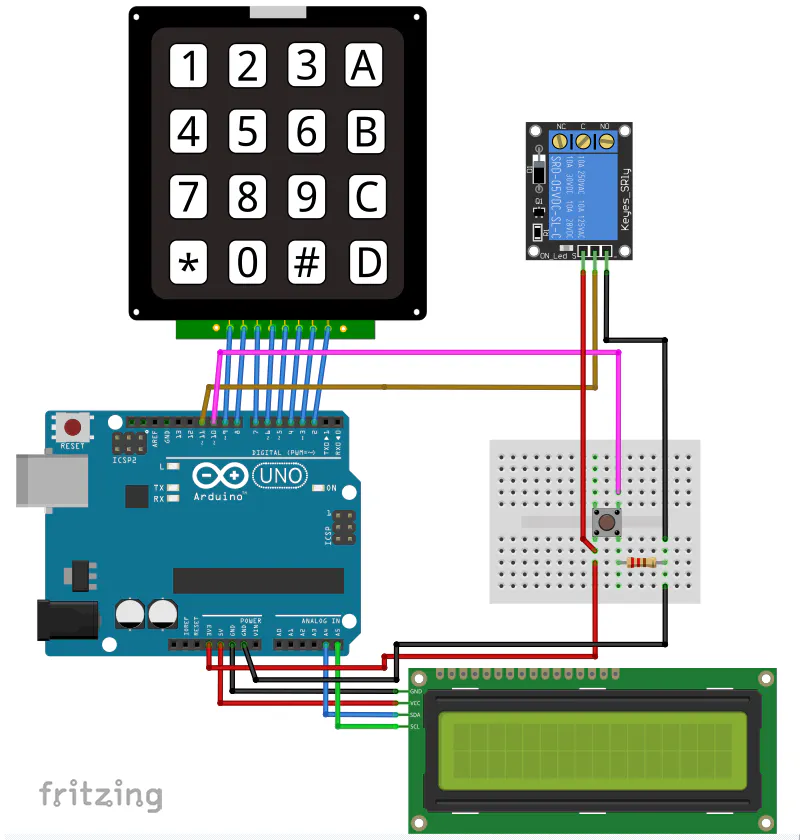# Keypad Door Lock with Changeable Code

Control a solenoid/relay or whatever your locking system, and change the passcode by the keypad only, no program reupload.

BeginnerFull instructions provided6,452## Things used in this project

### Hardware componentsArduino UNO & Genuino UNO
×1
×1
 Generic push button
×1
 LCD i²c
×1
 Linear solenoid lock
×1
 IRF510N MOSFET
×1
 1 Channel Relay
×1

### Hand tools and fabrication machines10 Pc. Jumper Wire Kit, 5 cm Long

## Schematics

### Mosfet + Solenoid wiring### Relay wiring## Code

Arduino
Remember to upload the code first then uncomment line 62 to 64 and reupload, it's done only once.
```/* This code works with 4x4 Keypad Matrix, LCD ic, IRF510N transistor and a push button
* It's a lock where the transistor drives a solenoid lock you can open either with correct code
* or by the push button from inside
* Code is stored in EEPROM
* Refer to www.surtrtech.com for more details
*/

#include <EEPROM.h>
#include <LiquidCrystal_I2C.h>

#define Solenoid 11             //Actually the Gate of the transistor that controls the solenoid
#define O_Button 10             //Push Button

#define I2C_ADDR 0x27           //I2C adress, you should use the code to scan the adress first (0x27) here
#define BACKLIGHT_PIN 3         // Declaring LCD Pins
#define En_pin 2
#define Rw_pin 1
#define Rs_pin 0
#define D4_pin 4
#define D5_pin 5
#define D6_pin 6
#define D7_pin 7

const byte numRows= 4;          //number of rows on the keypad
const byte numCols= 4;          //number of columns on the keypad

char keymap[numRows][numCols]=
{
{'1', '2', '3', 'A'},
{'4', '5', '6', 'B'},
{'7', '8', '9', 'C'},
{'*', '0', '#', 'D'}
};

char keypressed;                 //Where the keys are stored it changes very often
char code[]= {'6','6','0','1'};  //The default code, you can change it or make it a 'n' digits one

char code_buff1[sizeof(code)];  //Where the new key is stored
char code_buff2[sizeof(code)];  //Where the new key is stored again so it's compared to the previous one

short a=0,i=0,s=0,j=0;          //Variables used later

byte rowPins[numRows] = {9,8,7,6}; //Rows 0 to 3 //if you modify your pins you should modify this too
byte colPins[numCols]= {5,4,3,2}; //Columns 0 to 3

void setup()
{
lcd.begin (16,2);
lcd.setBacklightPin(BACKLIGHT_PIN,POSITIVE);
lcd.setBacklight(HIGH); //Lighting backlight
lcd.home ();
lcd.print("Standby");      //What's written on the LCD you can change

pinMode(Solenoid,OUTPUT);
pinMode(O_Button,INPUT);

//          for(i=0 ; i<sizeof(code);i++){        //When you upload the code the first time keep it commented
//            EEPROM.get(i, code[i]);             //Upload the code and change it to store it in the EEPROM
//             }                                  //Then uncomment this for loop and reupload the code (It's done only once)

}

void loop()
{

keypressed = myKeypad.getKey();               //Constantly waiting for a key to be pressed
if(keypressed == '*'){                      // * to open the lock
lcd.clear();
lcd.setCursor(0,0);
lcd.print("Enter code");            //Message to show
GetCode();                          //Getting code function
if(a==sizeof(code))           //The GetCode function assign a value to a (it's correct when it has the size of the code array)
OpenDoor();                   //Open lock function if code is correct
else{
lcd.clear();
lcd.print("Wrong");          //Message to print when the code is wrong
}
delay(2000);
lcd.clear();
lcd.print("Standby");             //Return to standby mode it's the message do display when waiting
}

if(keypressed == '#'){                  //To change the code it calls the changecode function
ChangeCode();
lcd.clear();
lcd.print("Standby");                 //When done it returns to standby mode
}

if(digitalRead(O_Button)==HIGH){      //Opening by the push button
digitalWrite(Solenoid,HIGH);
delay(3000);                        //Opens for 3s you can change
digitalWrite(Solenoid,LOW);

}

}

void GetCode(){                  //Getting code sequence
i=0;                      //All variables set to 0
a=0;
j=0;

while(keypressed != 'A'){                                     //The user press A to confirm the code otherwise he can keep typing
if(keypressed != NO_KEY && keypressed != 'A' ){       //If the char typed isn't A and neither "nothing"
lcd.setCursor(j,1);                                  //This to write "*" on the LCD whenever a key is pressed it's position is controlled by j
lcd.print("*");
j++;
if(keypressed == code[i]&& i<sizeof(code)){            //if the char typed is correct a and i increments to verify the next caracter
a++;                                              //Now I think maybe I should have use only a or i ... too lazy to test it -_-'
i++;
}
else
a--;                                               //if the character typed is wrong a decrements and cannot equal the size of code []
}
}
keypressed = NO_KEY;

}

void ChangeCode(){                      //Change code sequence
lcd.clear();
lcd.print("Changing code");
delay(1000);
lcd.clear();
lcd.print("Enter old code");
GetCode();                      //verify the old code first so you can change it

if(a==sizeof(code)){      //again verifying the a value
lcd.clear();
lcd.print("Changing code");
GetNewCode1();            //Get the new code
GetNewCode2();            //Get the new code again to confirm it
s=0;
for(i=0 ; i<sizeof(code) ; i++){     //Compare codes in array 1 and array 2 from two previous functions
if(code_buff1[i]==code_buff2[i])
s++;                                //again this how we verifiy, increment s whenever codes are matching
}
if(s==sizeof(code)){            //Correct is always the size of the array

for(i=0 ; i<sizeof(code) ; i++){
code[i]=code_buff2[i];         //the code array now receives the new code
EEPROM.put(i, code[i]);        //And stores it in the EEPROM

}
lcd.clear();
lcd.print("Code Changed");
delay(2000);
}
else{                         //In case the new codes aren't matching
lcd.clear();
lcd.print("Codes are not");
lcd.setCursor(0,1);
lcd.print("matching !!");
delay(2000);
}

}

else{                     //In case the old code is wrong you can't change it
lcd.clear();
lcd.print("Wrong");
delay(2000);
}
}

void GetNewCode1(){
i=0;
j=0;
lcd.clear();
lcd.print("Enter new code");   //tell the user to enter the new code and press A
lcd.setCursor(0,1);
lcd.print("and press A");
delay(2000);
lcd.clear();
lcd.setCursor(0,1);
lcd.print("and press A");     //Press A keep showing while the top row print ***

while(keypressed != 'A'){            //A to confirm and quits the loop
if(keypressed != NO_KEY && keypressed != 'A' ){
lcd.setCursor(j,0);
lcd.print("*");               //On the new code you can show * as I did or change it to keypressed to show the keys
code_buff1[i]=keypressed;     //Store caracters in the array
i++;
j++;
}
}
keypressed = NO_KEY;
}

void GetNewCode2(){                         //This is exactly like the GetNewCode1 function but this time the code is stored in another array
i=0;
j=0;

lcd.clear();
lcd.print("Confirm code");
lcd.setCursor(0,1);
lcd.print("and press A");
delay(3000);
lcd.clear();
lcd.setCursor(0,1);
lcd.print("and press A");

while(keypressed != 'A'){
if(keypressed != NO_KEY && keypressed != 'A' ){
lcd.setCursor(j,0);
lcd.print("*");
code_buff2[i]=keypressed;
i++;
j++;
}
}
keypressed = NO_KEY;
}

void OpenDoor(){             //Lock opening function open for 3s
lcd.clear();
lcd.print("Welcome");       //With a message printed
digitalWrite(Solenoid,HIGH);
delay(3000);
digitalWrite(Solenoid,LOW);
}
```

Arduino
Remember to upload the code first then uncomment line 62 to 64 and reupload, it's done only once.
```/* This code works with 4x4 Keypad Matrix, LCD ic, relay module and a push button
* It's a lock where the relay can control a lock you can open either with correct code
* or by the push button from inside
* Code is stored in EEPROM
* Refer to www.surtrtech.com for more details
*/

#include <EEPROM.h>
#include <LiquidCrystal_I2C.h>

#define Relay 11                //Controls the Relay
#define O_Button 10             //Push Button

#define I2C_ADDR 0x27           //I2C adress, you should use the code to scan the adress first (0x27) here
#define BACKLIGHT_PIN 3         // Declaring LCD Pins
#define En_pin 2
#define Rw_pin 1
#define Rs_pin 0
#define D4_pin 4
#define D5_pin 5
#define D6_pin 6
#define D7_pin 7

const byte numRows= 4;          //number of rows on the keypad
const byte numCols= 4;          //number of columns on the keypad

char keymap[numRows][numCols]=
{
{'1', '2', '3', 'A'},
{'4', '5', '6', 'B'},
{'7', '8', '9', 'C'},
{'*', '0', '#', 'D'}
};

char keypressed;                 //Where the keys are stored it changes very often
char code[]= {'6','6','0','1'};  //The default code, you can change it or make it a 'n' digits one

char code_buff1[sizeof(code)];  //Where the new key is stored
char code_buff2[sizeof(code)];  //Where the new key is stored again so it's compared to the previous one

short a=0,i=0,s=0,j=0;          //Variables used later

byte rowPins[numRows] = {9,8,7,6}; //Rows 0 to 3 //if you modify your pins you should modify this too
byte colPins[numCols]= {5,4,3,2}; //Columns 0 to 3

void setup()
{
lcd.begin (16,2);
lcd.setBacklightPin(BACKLIGHT_PIN,POSITIVE);
lcd.setBacklight(HIGH); //Lighting backlight
lcd.home ();
lcd.print("Standby");      //What's written on the LCD you can change

pinMode(Relay,OUTPUT);
pinMode(O_Button,INPUT);

//          for(i=0 ; i<sizeof(code);i++){        //When you upload the code the first time keep it commented
//            EEPROM.get(i, code[i]);             //Upload the code and change it to store it in the EEPROM
//             }                                  //Then uncomment this for loop and reupload the code (It's done only once)

}

void loop()
{

keypressed = myKeypad.getKey();               //Constantly waiting for a key to be pressed
if(keypressed == '*'){                      // * to open the lock
lcd.clear();
lcd.setCursor(0,0);
lcd.print("Enter code");            //Message to show
GetCode();                          //Getting code function
if(a==sizeof(code))           //The GetCode function assign a value to a (it's correct when it has the size of the code array)
OpenDoor();                   //Open lock function if code is correct
else{
lcd.clear();
lcd.print("Wrong");          //Message to print when the code is wrong
}
delay(2000);
lcd.clear();
lcd.print("Standby");             //Return to standby mode it's the message do display when waiting
}

if(keypressed == '#'){                  //To change the code it calls the changecode function
ChangeCode();
lcd.clear();
lcd.print("Standby");                 //When done it returns to standby mode
}

if(digitalRead(O_Button)==HIGH){      //Opening by the push button
digitalWrite(Relay,LOW);
delay(3000);                        //Opens for 3s you can change
digitalWrite(Relay,HIGH);

}

}

void GetCode(){                  //Getting code sequence
i=0;                      //All variables set to 0
a=0;
j=0;

while(keypressed != 'A'){                                     //The user press A to confirm the code otherwise he can keep typing
if(keypressed != NO_KEY && keypressed != 'A' ){       //If the char typed isn't A and neither "nothing"
lcd.setCursor(j,1);                                  //This to write "*" on the LCD whenever a key is pressed it's position is controlled by j
lcd.print("*");
j++;
if(keypressed == code[i]&& i<sizeof(code)){            //if the char typed is correct a and i increments to verify the next caracter
a++;                                              //Now I think maybe I should have use only a or i ... too lazy to test it -_-'
i++;
}
else
a--;                                               //if the character typed is wrong a decrements and cannot equal the size of code []
}
}
keypressed = NO_KEY;

}

void ChangeCode(){                      //Change code sequence
lcd.clear();
lcd.print("Changing code");
delay(1000);
lcd.clear();
lcd.print("Enter old code");
GetCode();                      //verify the old code first so you can change it

if(a==sizeof(code)){      //again verifying the a value
lcd.clear();
lcd.print("Changing code");
GetNewCode1();            //Get the new code
GetNewCode2();            //Get the new code again to confirm it
s=0;
for(i=0 ; i<sizeof(code) ; i++){     //Compare codes in array 1 and array 2 from two previous functions
if(code_buff1[i]==code_buff2[i])
s++;                                //again this how we verifiy, increment s whenever codes are matching
}
if(s==sizeof(code)){            //Correct is always the size of the array

for(i=0 ; i<sizeof(code) ; i++){
code[i]=code_buff2[i];         //the code array now receives the new code
EEPROM.put(i, code[i]);        //And stores it in the EEPROM

}
lcd.clear();
lcd.print("Code Changed");
delay(2000);
}
else{                         //In case the new codes aren't matching
lcd.clear();
lcd.print("Codes are not");
lcd.setCursor(0,1);
lcd.print("matching !!");
delay(2000);
}

}

else{                     //In case the old code is wrong you can't change it
lcd.clear();
lcd.print("Wrong");
delay(2000);
}
}

void GetNewCode1(){
i=0;
j=0;
lcd.clear();
lcd.print("Enter new code");   //tell the user to enter the new code and press A
lcd.setCursor(0,1);
lcd.print("and press A");
delay(2000);
lcd.clear();
lcd.setCursor(0,1);
lcd.print("and press A");     //Press A keep showing while the top row print ***

while(keypressed != 'A'){            //A to confirm and quits the loop
if(keypressed != NO_KEY && keypressed != 'A' ){
lcd.setCursor(j,0);
lcd.print("*");               //On the new code you can show * as I did or change it to keypressed to show the keys
code_buff1[i]=keypressed;     //Store caracters in the array
i++;
j++;
}
}
keypressed = NO_KEY;
}

void GetNewCode2(){                         //This is exactly like the GetNewCode1 function but this time the code is stored in another array
i=0;
j=0;

lcd.clear();
lcd.print("Confirm code");
lcd.setCursor(0,1);
lcd.print("and press A");
delay(3000);
lcd.clear();
lcd.setCursor(0,1);
lcd.print("and press A");

while(keypressed != 'A'){
if(keypressed != NO_KEY && keypressed != 'A' ){
lcd.setCursor(j,0);
lcd.print("*");
code_buff2[i]=keypressed;
i++;
j++;
}
}
keypressed = NO_KEY;
}

void OpenDoor(){             //Lock opening function open for 3s
lcd.clear();
lcd.print("Welcome");       //With a message printed
digitalWrite(Relay,LOW);
delay(3000);
digitalWrite(Relay,HIGH);
}
```

## Credits

### SurtrTech

6 projects • 92 followers
YT Channel, run by Automation and Electrical Engineer, Electronics amateur.